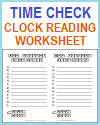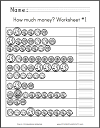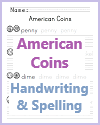Grade 2 Math - Measurement and Data Worksheets | Student Handouts

CCSS.MATH.CONTENT.2.MD.A.1 - Measure the length of an object by selecting and using appropriate tools such as rulers, yardsticks, meter sticks, and measuring tapes.

CCSS.MATH.CONTENT.2.MD.A.2 - Measure the length of an object twice, using length units of different lengths for the two measurements; describe how the two measurements relate to the size of the unit chosen.

CCSS.MATH.CONTENT.2.MD.A.3 - Estimate lengths using units of inches, feet, centimeters, and meters.

CCSS.MATH.CONTENT.2.MD.A.4 - Measure to determine how much longer one object is than another, expressing the length difference in terms of a standard length unit.

CCSS.MATH.CONTENT.2.MD.B.5 - Use addition and subtraction within 100 to solve word problems involving lengths that are given in the same units, e.g., by using drawings (such as drawings of rulers) and equations with a symbol for the unknown number to represent the problem.

CCSS.MATH.CONTENT.2.MD.B.6 - Represent whole numbers as lengths from 0 on a number line diagram with equally spaced points corresponding to the numbers 0, 1, 2, ..., and represent whole-number sums and differences within 100 on a number line diagram.

CCSS.MATH.CONTENT.2.MD.C.7 - Tell and write time from analog and digital clocks to the nearest five minutes, using a.m. and p.m.Time Check Clock-reading Handout
CCSS.MATH.CONTENT.2.MD.C.8 - Solve word problems involving dollar bills, quarters, dimes, nickels, and pennies, using \$ and ¢ symbols appropriately. Example: If you have 2 dimes and 3 pennies, how many cents do you have?Counting Money Worksheets American Coins Identification and Spelling Worksheet

CCSS.MATH.CONTENT.2.MD.D.9 - Generate measurement data by measuring lengths of several objects to the nearest whole unit, or by making repeated measurements of the same object. Show the measurements by making a line plot, where the horizontal scale is marked off in whole-number units.

CCSS.MATH.CONTENT.2.MD.D.10 - Draw a picture graph and a bar graph (with single-unit scale) to represent a data set with up to four categories. Solve simple put-together, take-apart, and compare problems1 using information presented in a bar graph.